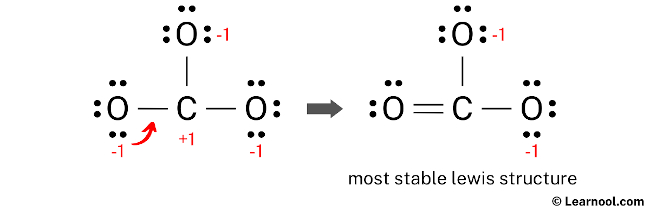# CO32- Lewis structure

The CO32- Lewis structure represents a carbonate ion consisting of one carbon atom and three oxygen atoms. The carbon atom forms one double bond and two single bonds with the three oxygen atoms. Among these, the oxygen atom that shares a double bond has two lone pairs, while the two oxygen atoms that share single bonds have three lone pairs each. Additionally, the two oxygen atoms attached by single bonds carry a negative charge of -1.

To draw an accurate CO32- Lewis structure, start by sketching a rough structure of the molecule and indicating the locations of any lone pairs on the atoms. Then, determine the formal charges on the atoms and minimize them by converting any available lone pairs, repeating this step until all charges are minimized. Following these steps results in a stable CO32- Lewis structure that depicts the arrangement of atoms and electrons in the molecule.

Contents

## Steps

### Sketch the structure

The first step in drawing the CO32- Lewis structure is to determine the total number of valence electrons in the molecule. This can be calculated by multiplying the valence electrons of each atom. Carbon is located in group 14 of the periodic table and has four valence electrons, while oxygen, belonging to group 16, has six valence electrons. In CO32-, which consists of one carbon atom and three oxygen atoms, the total valence electrons can be calculated as follows: the valence electrons of one carbon atom are 4 × 1 = 4, and the valence electrons of three oxygen atoms are 6 × 3 = 18. Additionally, since CO32- carries a negative (-2) charge, two more electrons need to be added, bringing the total number of valence electrons to 24.

The second step in drawing the CO32- Lewis structure is to determine the total number of electron pairs in the molecule. This is done by dividing the total number of valence electrons by two. In the case of CO32-, which has a total of 24 valence electrons, the number of electron pairs is calculated as 24 ÷ 2 = 12. This means that there are a total of 12 electron pairs in the CO32- molecule that need to be distributed among the atoms to form a stable Lewis structure.

Once the total number of electron pairs has been determined, the next step in drawing the CO32- Lewis structure is to identify the central atom. The least electronegative atom is typically placed in the center, and in the case of CO32-, carbon is less electronegative than oxygen. Therefore, carbon should be placed in the center and the oxygen atoms should be arranged around it. With this in mind, a rough sketch of the CO32- Lewis structure can be drawn.

### Indicate lone pair

After sketching the CO32- Lewis structure, the next step is to indicate the location of lone pairs on the atoms. Based on the calculation, it has been determined that there are a total of 12 electron pairs in the CO32- molecule. Since three C – O bonds, utilizing six electron pairs, have already been marked in the sketch, the remaining nine electron pairs need to be indicated as lone pairs on the atoms.

It’s worth noting that both carbon and oxygen are period 2 elements, which means they can accommodate a maximum of eight electrons in their outermost shell.

To ensure the correct distribution of electrons and to satisfy the octet rule, it is important to start indicating the lone pairs from the outer atoms. In the case of CO32-, the outer atoms are oxygen. Therefore, each oxygen atom must have three lone pairs, while the central carbon atom should not have any lone pair as all nine electron pairs are utilized. Carefully mark the lone pairs on the sketch according to this arrangement to create a stable Lewis structure.

### Assign formal charge

The formal charges for the atoms in CO32- can be calculated using the following formula: Formal charge = valence electrons – nonbonding electrons – ½ bonding electrons. For the carbon atom, the formal charge can be calculated as 4 – 0 – ½ (6) = +1. Similarly, for each oxygen atom, the formal charge can be calculated as 6 – 6 – ½ (2) = -1.

 Atom Valence electron Nonbonding electron Bonding electron Formal charge Carbon 4 0 6 +1 Oxygen 6 6 2 -1

Once the formal charges have been determined, mark them on the sketch of the molecule accordingly to indicate the charge on each atom.

The current Lewis structure of CO32- is not stable as both carbon and oxygen atoms have charges. In order to stabilize the structure, it is necessary to reduce the charges by converting lone pairs to bonds.

### Minimize formal charge

To minimize the formal charges in the CO32- molecule, it is necessary to create a new C – O bond by converting a lone pair of one of the oxygen atoms to a bonding pair with the central carbon atom.Lone pair of left oxygen is converted, and got the most stable Lewis structure of CO32-

After minimizing the formal charges, the CO32- Lewis structure has the central carbon atom and the outer oxygen atoms satisfying the octet rule. However, the two oxygen atoms still have a negative (-1) charge. This is acceptable since the most electronegative atom typically carries a negative charge in a stable Lewis structure. Moreover, this structure is more stable than previous ones. Therefore, this arrangement represents the most stable Lewis structure for CO32-. Finally, to reflect the molecule’s overall charge of negative (-2), denote the charge outside the brackets in the structure.